# GitHub in 2013: Event Types and Commits

In this IPython notebook I present a brief visual overview of GitHub event types in 2013 based on data obtained from the GitHub Archive.

The GitHub Archive makes data available as gzipped files that each contain a stream of JSON encoded GitHub events. There is one archive file for each hour of each day. I downloaded all the files availble for 2013 (9 files/hours are missing) and pre-processed them to create the CSV files used here. The pre-processing steps won't be covered in this notebook.

The source code of this notebook and the data files used are available in this GitHub repository.

## Preliminaries

First load the necessary packages, set some matplotlib configuration parameters and create a list of short weekday names used for labels later on.

In :
```import itertools

import pandas as pd
import numpy as np
import matplotlib.pyplot as plt

plt.rcParams['axes.grid'] = False
plt.rcParams['grid.linewidth'] = 0

weekdays_short = ['Mon', 'Tue', 'Wed', 'Thu', 'Fri', 'Sat', 'Sun']
```

Read the event counts by day for 2013 from a CSV file, set the first column as the index and specify, that it is a date. Also remove `Event` from the column labels and add a column with the total number of events.

In :
```df_events = pd.read_csv('csv/githubarchive/2013/event_counts_by_day.csv', index_col=0, parse_dates=)
df_events.columns = df_events.columns.map(lambda x: x.replace('Event', ''))
```
Out:
CommitComment Create Delete Download Follow Fork Gist Gollum IssueComment Issues Member Public PullRequest PullRequestReviewComment Push Release Watch
2013-01-01 878 10770 618 8 2009 3712 449 2046 9450 7176 441 87 2472 396 47997 NaN 9662
2013-01-02 1491 16367 1088 15 3175 5765 779 2738 13098 8067 792 140 5088 1246 73343 NaN 15956
2013-01-03 1744 29179 1112 240 4606 6490 936 3117 13720 8033 895 159 5674 1410 78656 NaN 15835
2013-01-04 1606 17347 1168 106 4210 6063 763 3268 13955 9859 798 140 5727 1260 75899 NaN 15683
2013-01-05 983 13919 808 20 2771 4829 707 2345 8804 5928 614 97 3303 720 59961 NaN 12232

## Events by Type

Let's first look at how frequent the different event types are in total.

In :
```event_sums = df_events.sum(axis=0)
event_sums.sort()
event_sums.plot(kind='barh', figsize=(12, 8))
plt.show()
```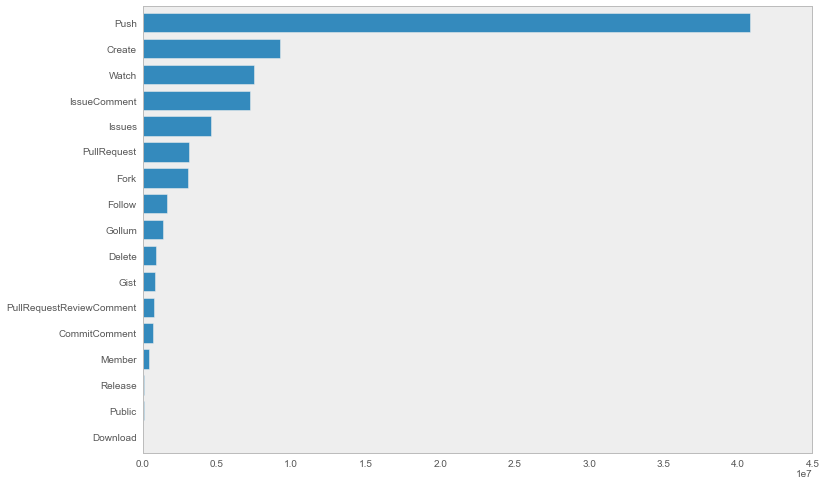Unsurprisingly pushes occur most often, in fact almost as often as all other events combined.

In :
```last = len(event_sums) - 1
sums = [int(event_sums[1: last].sum()), int(event_sums[last])]

fig = pd.Series(sums, index=('All but Pushes', 'Pushes')).plot(kind='bar')
fig.set_xticklabels(('All but Pushes', 'Pushes'), rotation=0)
fig.annotate(sums, [0, sums], ha='left', textcoords='offset points', xytext=(75, 5))
fig.annotate(sums, [0, sums], ha='left', textcoords='offset points', xytext=(225, 5))
plt.show()
```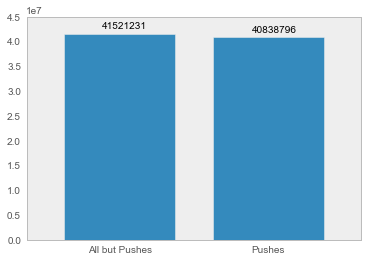## Event Timelines

Now let's look at how the 17 different GitHub event types evolved in 2013 by plotting a line graph for each type with time on the x-axes and the total number of events aggregated by day on the y-axes. I also add a column with the total number of events to have an even number of data series to plot.

In :
```df_events['Total'] = df_events.sum(axis=1)

fig, axes = plt.subplots(nrows=9, ncols=2)
fig.suptitle('GitHub event type timelines for 2013', y=1.01, fontsize=14)
fig.set_figheight(26)
fig.set_figwidth(14)

cols = df_events.columns
lencols = len(cols)

for idx, coords in enumerate(itertools.product(range(9), range(2))):
if idx < lencols:
ax = axes[coords, coords]
ax.set_title(cols[idx])
df_events[cols[idx]].plot(ax=ax)
ax.set_xlabel('', visible=False)
fig.tight_layout()
```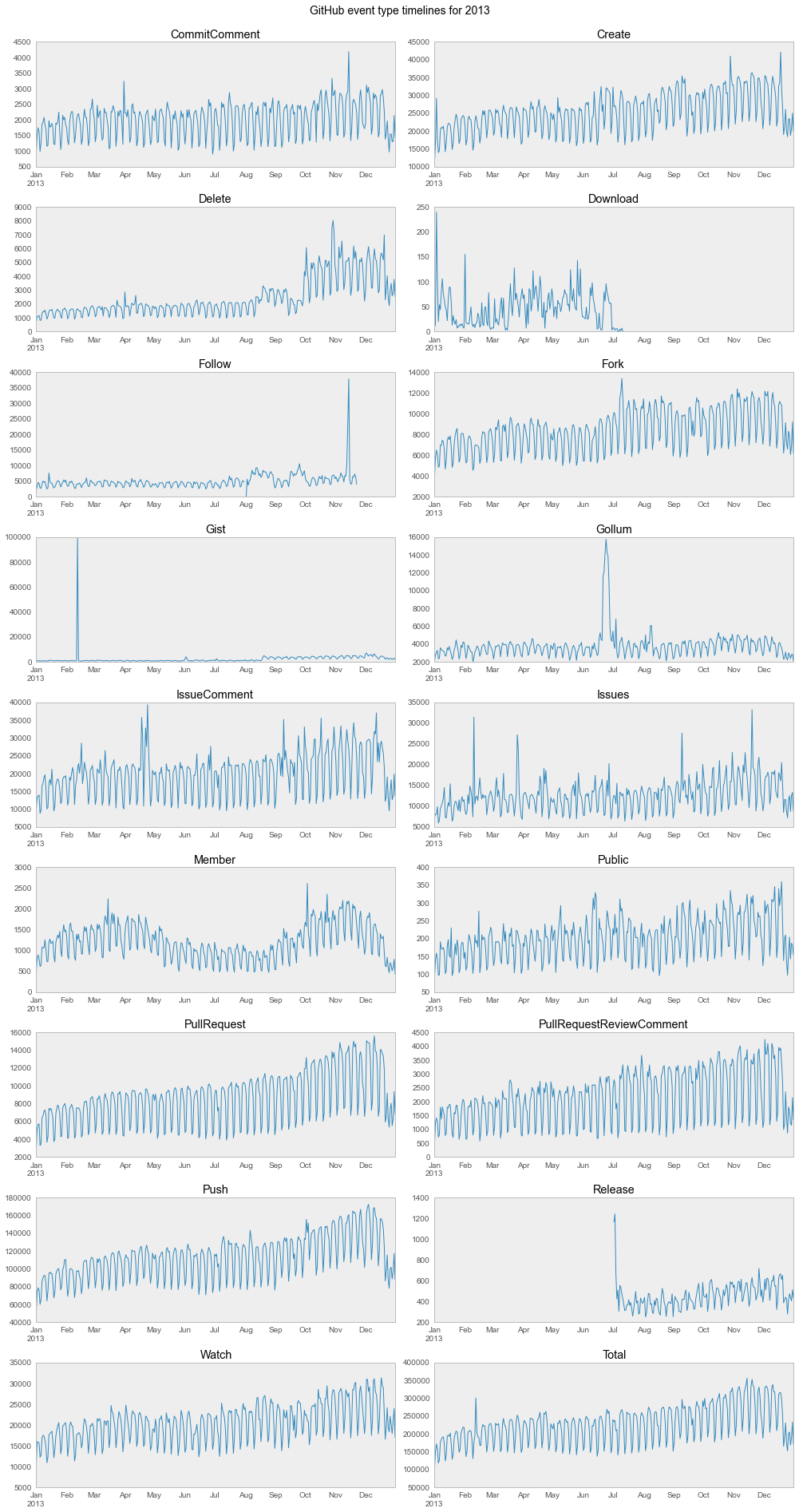We can clearly see that GitHub has grown in 2013 and one pattern that is common to all graphs are increases at the start of the week and drop-offs towards the end.

`Download` and `Follow` events were obviously removed from the public timeline in the past year, whereas `Release` events were introduced in the beginning of July.

In :
```df_events['Release'].dropna().head()
```
Out:
```2013-07-02    1165
2013-07-03    1241
2013-07-04     663
2013-07-05     423
2013-07-06     510
Name: Release, dtype: float64
```

There are extreme spikes in some of the graphs, for example at the end of November in the `Follow` events. When exactly does this spike occur?

In :
```df_events[['Follow']].dropna().sort('Follow').tail()
```
Out:
Follow
2013-08-13 9322
2013-11-12 10193
2013-09-25 10598
2013-11-13 23332
2013-11-14 37823

The events `DataFrame` does not allow us to dig deeper, to see what might have caused this spike, but I'll keep this in mind when looking at `Follow` events in a future notebook. Update: the follow events notebook is published.

## Event Types by Weekday

First add a weekday column to the data frame, which is very easy since the index contains a date. Then group by weekday and aggregate the grouped events calculating the mean and median values.

In :
```# 0 = monday
df_events['Weekday'] = df_events.index.weekday
grouped = df_events.groupby('Weekday').agg([np.mean, np.median])
```

The next step is to plot a bar chart for each event type, showing the distributions of mean and median event counts per weekday.

In :
```keys = grouped.keys()
cols = grouped.columns
lencols = len(cols)

fig, axes = plt.subplots(nrows=6, ncols=3)
fig.suptitle('Mean and median frequencies of GitHub event types per weekday', y=1.01, fontsize=14)
fig.set_figheight(20)
fig.set_figwidth(14)

for idx, coords in enumerate(itertools.product(range(6), range(3))):
if idx < lencols:
ax = axes[coords, coords]
start = idx * 2
grouped[[start, start + 1]].plot(ax=ax, kind='bar', legend=False)
ax.set_title(cols[start])
ax.set_xticklabels(weekdays_short, rotation=0)
ax.set_xlabel('', visible=False)
fig.tight_layout()
```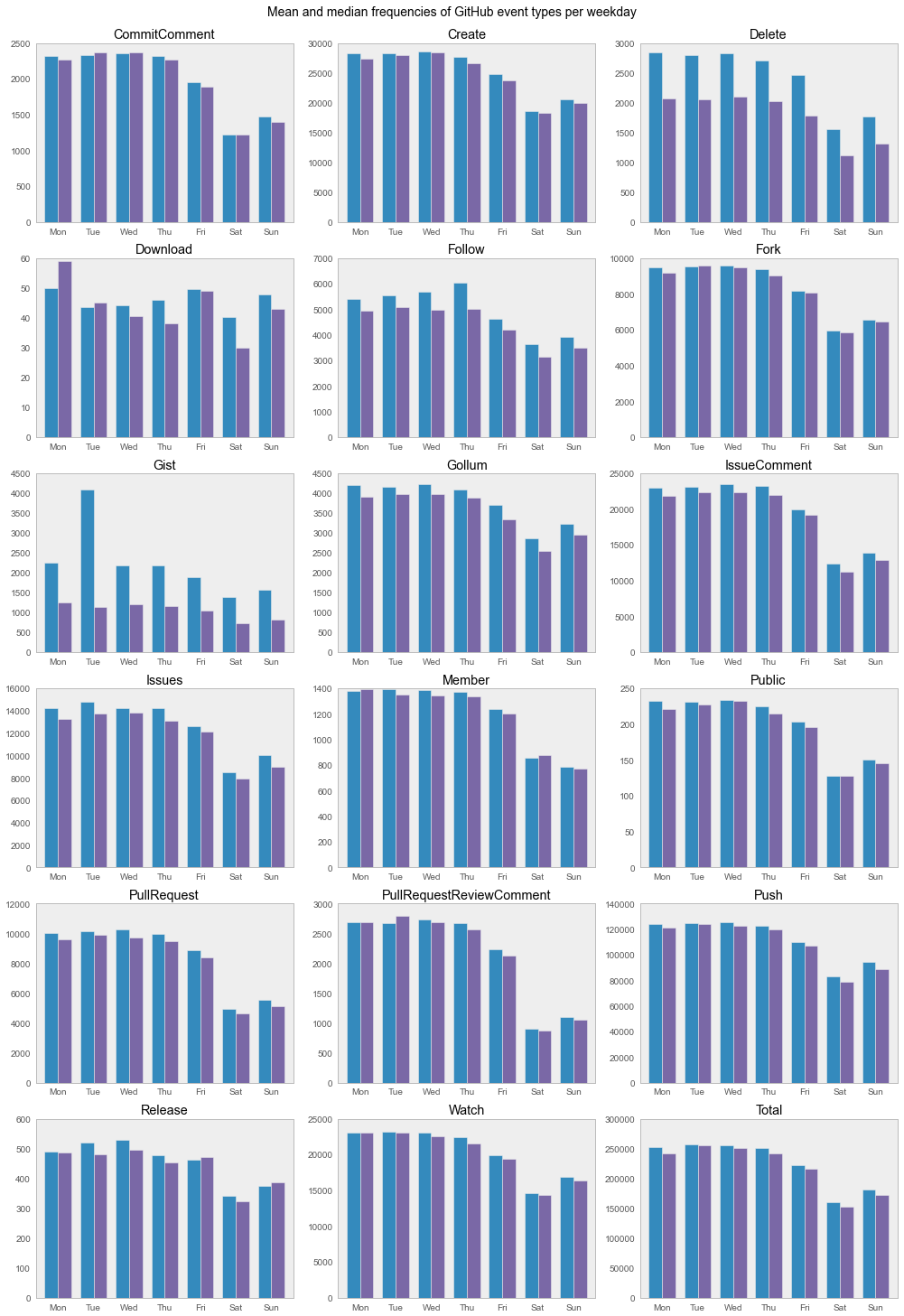I haven't figured out a good way to add just a single legend for the whole multi-plot, which shows that blue is mean and purple median. If you have an idea let me know in the comments below.

For most event types these graphs confirm the weekend drop-offs we already saw in the timelines, with more activity on Sundays than on Saturdays. One notable exception are `Download` events, where Sunday is on average the 3rd most active day of the week.

Moreover, there are considerable differences between mean and median values for `Delete` and `Gist` events. Looking back at the `Gist` timeline we see a huge spike, which must have happened on a Tuesday.

In :
```df_events[['Gist', 'Weekday']].dropna().sort('Gist').tail(3)
```
Out:
Gist Weekday
2013-12-01 6448 6
2013-12-02 7070 0
2013-02-12 99435 1

Again, we cannot figure out what happened that day using the current dataset, but it's something to look at more deeply in one of the next posts.

## Commits

The GitHub API doesn't have a dedicated `Commit` event type, instead commits are contained in push events. Data for push events per day is aggregated in the CSV file loaded next. The number of commits per day is kept in the `Event Size` column.

In :
```df_pushes = pd.read_csv('csv/githubarchive/2013/pushes_by_day.csv', index_col=0, parse_dates=)
```

Since the number of commits per push event varies, let's look at the ratios of commits to pushes over the course of the year.

In :
```df_pushes['Commit Push Ratio'] = df_pushes['Event Size'] / df_pushes['Event Count'].astype(float)
df_pushes['Commit Push Ratio'].plot(figsize=(14, 10), title='Ratios of commits per push over time')
plt.show()
```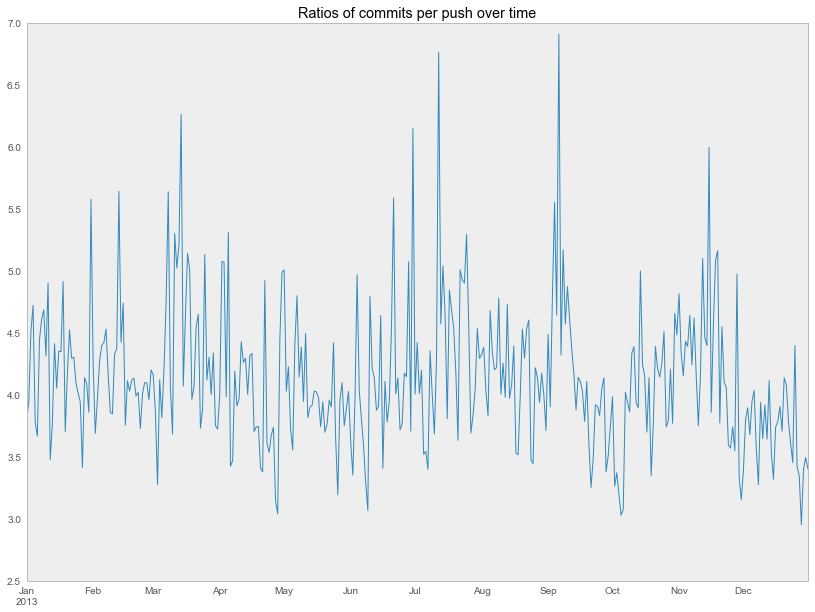There is quite some variation here, more than I had expected. A possible explanation is that some pushes contain a very high number of commits, e. g. when a feature branch is pushed for the first time, and some a very low number, which would be the case for hotfixes.

## Commits over Time

The last graph in this overview is commits over time. This time not as a line chart, but using a visualization similar to the one you see on GitHub user pages for their contributions.

To do so we group over weekday and week number summing up the commit counts (`Event Size`) and store them in a list of lists, one for each weekday containing the commit counts for each week number of the year 2013.

In :
```df_pushes['Week'] = df_pushes.index.week
df_pushes['Weekday'] = df_pushes.index.weekday
grouped = df_pushes.groupby(['Weekday', 'Week']).sum()
image = [grouped['Event Size'][i] for i in range(7)]
```

A few things to note about the following plot are setting the spines visibility to `False` to not show lines around the plot and explicitly setting the ticks, the y-axis ticks to the short weekday names and the x-axis ticks to the numbers from 1 to 52. Otherwise the x-ticks would range from 0 to 51 corresponding to the list indices of the image data passed to the `imshow` method.

In :
```fig, ax = plt.subplots(figsize=(16, 9))
ax.imshow(image, cmap=plt.cm.Greens, interpolation='nearest')
ax.set_title('Commits by weekday and week')

for pos in ['top', 'right', 'bottom', 'left']:
ax.spines[pos].set_visible(False)

plt.yticks(range(7), weekdays_short)
plt.xticks(range(52), range(1, 53))
plt.show()
```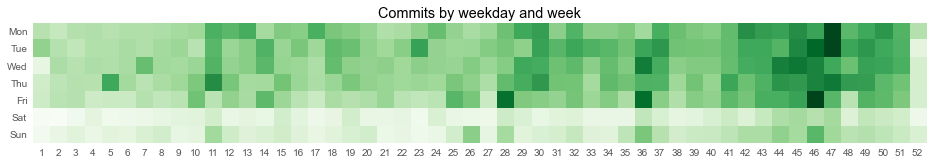Using this type of visualization, the increase of commits over time is not as clearly visible as in the line graphs above, but it allows to determine days with unusual activity, especially days with very high numbers of commits, that are worth exploring further.

## Summary

In this notebook I presented an overview of the different types of events that occurred on GitHub in 2013 focusing on the evolution of event types over time and their distribution across weekdays.

A few of the things we could see are that GitHub grew in the past year and that there are some days with extremely high activity for some event types, which asks for further investigation.

This is just the tip of the iceberg, there is a lot more information contained in the GitHub Archive data, that I'm going to present in other GitHub Archive posts.

If you have questions that could be answered from this data, feel free to ask them in the comments.

### Featured Merch

This post was written by Ramiro Gómez (@yaph) and published on . Subscribe to the Geeksta RSS feed to be informed about new posts.

Disclosure: External links on this website may contain affiliate IDs, which means that I earn a commission if you make a purchase using these links. This allows me to offer hopefully valuable content for free while keeping this website sustainable. For more information, please see the disclosure section on the about page.

Share post: